# Cluster Analysis

#### 4/13/2020

# load python
library(reticulate)
use_condaenv("my_ml")
# load packages
import pandas as pd
import matplotlib.pyplot as plt
import numpy as np
from sklearn.cluster import KMeans
import seaborn as sns; sns.set()
from scipy.spatial import distance
from sklearn.metrics import silhouette_score
from sklearn.mixture import GaussianMixture

# 1 Introduction to Cluster Analysis

Cluster analysis is a form of unsupervised learning which aims to discover and explore the underlying structure in the data. The crux of a cluster analysis algorithm is distance metrics: the way you measure similarity or distance between observations. Unsupervised learning is often used in situations where you do not have labelled data (perhaps it is expensive) or when you might not know the correct values for some of your data and therefore, you might want to evaluate its underlying structure.

# 2 Principles of Cluster Analysis

Cluster analysis’ basic principle is that you want to find the natural groupings or clusters in your data set. Typically, the correct groupings are not known a priori and really what makes cluster analysis successful is when you can identify clusters such that observations within the cluster are more similar than observations that are in different clusters.

# 3 Measuring Similarity

The distance function, or the way we measure similarity in a clustering algorithm, determines how effective clustering is going to be on your data set. Since the goal of cluster analysis is to identify groups in data, one of the key questions is what makes a group a group. Intuitively, a group should be comprised of similar items and different groups should be comprised of dissimilar items. Similarity/dissimilarity is operationalized by distance metrics and we tend to measure distance based on the types of variables (i.e., categorical, continuous, etc) that we have.

There are five common distance algorithms that we might choose from:

1. Euclidean (L2): Euclidean distance is the square root of the sum of the square distances between two points. Euclidean distance is sometimes called $$L2$$ norm or $$L2$$ distance because we are taking values to the second power and taking the square root of the sum of squares.

2. Manhattan (L1): We could also take the $$n$$ root of the sum of the $$n$$th power. And so when $$n$$ equals 1, we call that the L1 norm which is the Manhattan distance. In other words, Manhattan distance is the raw sum of distances across all dimensions.

3. Chebyshev (L$$\infty$$): It takes the max of distances across any dimension. It says that two points are going to be very dissimilar if on any one of the k-dimensions they are very dissimilar. This stands in contrast to Manhattan distance which balances differences across all dimensions relatively evenly.

4. L($$n$$): Following suit, this algorithm takes the $$n$$ root of the sum of the $$n$$th power. It increases the effect of outliers on measuring distance between points .

5. Levenshtein: The Levenshtein or edit distance measures the distance between words. Distance is measured by the number of character substitutions it would take to get from one word to another.

Distance metrics must satisfy the following properties:

1. It is non-negative, so the distance between two points can never be less than 0.

2. It should be symmetric so that the distance between $$p$$ and $$q$$ is the same as the distance between $$q$$ and $$p$$.

3. And it should satisfy the triangle inequality. So the distance between $$a$$ and $$c$$ should be greater than the distance between $$a$$ and $$b$$ and $$b$$ and $$c$$.

Lastly, when using distance metrics, we often want to standardize our data (i.e., subtract the mean and divide by its standard deviation) as this makes it sure that the spread along any given dimension is roughly the same. This is of unless of course we a priori reason to think that one dimension should matter more than another in terms of how different the resultant clusters are.

# 4 Clustering in Practice: K-Means

K-means clustering is likely the most common form of clustering and one of the most common forms of unsupervised learning. K-means clustering takes our $$n$$ observations and groups them into a set of $$k$$ clusters. This means that before running our algorithm, we need to identify, a prior how many clusters we want to end up with which equals the value of $$k$$. Each of the $$k$$ clusters will get one centroid ($$\mu_{k}$$) – the geometric mean of the cluster in your high-dimensional subspace.

Following the discussion above, our first step is to pick a distance function. Our second step is to identify our loss function (i.e., the measure of fit of our clusters to the data). For Euclidean distance, our loss function is the sum of the square distances between all data points and the cluster to which they’re assigned.

This means that we:

1. Assign our centroids $$\mu_{k}$$.

2. Assign each observation to a centroid.

3. Realign our centroids following our minimization of our loss function.

4. Repeat until all centroids converged on a local minimum.

## 4.1 Clustering in Practice: K-Means

# Load the data
X, y = iris.data, iris.feature_names

# shuffle the data
shuffle = np.random.permutation(np.arange(X.shape))
X = X[shuffle]

# scale X
X = (X - X.mean()) / X.std()

There are several diagnostics that we can use to help us determine the optimal number of clusters. The first is AIC and BIC and the second is the silhouette score.

### 4.1.1 Quantity of Interest: AIC and BIC

If we look at finding the optimal number of clusters as a likelihood-maximization problem, we can use Information Criteria (BIC and AIC) to estimate when we can stop increasing the number: if we are not adding new information by adding one more cluster, we are done.

By evaluating our BIC scores below, we can see that the optimal number of clusters is 3, which we know is the case given the famous iris data set.

def computeBIC (clstrs, X):
n = X.shape
m = X.shape
k = len(clstrs.cluster_centers_)
Dw = np.power(clstrs.inertia_, 2)
Db = 0
for cc0 in range (k):
for cc1 in range (k):
if not cc0 == cc1:
Db = Db + distance.euclidean (clstrs.cluster_centers_[cc0], clstrs.cluster_centers_[cc1])
D = np.sqrt (Dw + np.power(Db, 2))
BIC = D + np.log(n)*m*k
AIC = D + 2*m*k
return BIC, AIC

for ff in np.arange(X.shape):
myX = X[:,ff].reshape(len (X[:,ff]), 1)
print ("Feature: %s" % (y[ff]))

for nn in range (1,6):
km = KMeans(n_clusters=nn, n_init=1, random_state=42)
clstrs = km.fit(myX)
bic, aic = computeBIC(clstrs, myX)
print ("Feature: %s. Number of clusters = %d.  BIC = %.3f. AIC = %.3f" % (y[ff], nn, bic, aic))
## Feature: sepal length (cm)
## Feature: sepal length (cm). Number of clusters = 1.  BIC = 26.224. AIC = 326.224
## Feature: sepal length (cm). Number of clusters = 2.  BIC = 8.064. AIC = 608.064
## Feature: sepal length (cm). Number of clusters = 3.  BIC = 5.782. AIC = 905.782
## Feature: sepal length (cm). Number of clusters = 4.  BIC = 8.813. AIC = 1208.813
## Feature: sepal length (cm). Number of clusters = 5.  BIC = 12.987. AIC = 1512.987
## Feature: sepal width (cm)
## Feature: sepal width (cm). Number of clusters = 1.  BIC = 7.266. AIC = 307.266
## Feature: sepal width (cm). Number of clusters = 2.  BIC = 2.931. AIC = 602.931
## Feature: sepal width (cm). Number of clusters = 3.  BIC = 2.815. AIC = 902.815
## Feature: sepal width (cm). Number of clusters = 4.  BIC = 4.760. AIC = 1204.760
## Feature: sepal width (cm). Number of clusters = 5.  BIC = 8.313. AIC = 1508.313
## Feature: petal length (cm)
## Feature: petal length (cm). Number of clusters = 1.  BIC = 119.178. AIC = 419.178
## Feature: petal length (cm). Number of clusters = 2.  BIC = 17.697. AIC = 617.697
## Feature: petal length (cm). Number of clusters = 3.  BIC = 10.974. AIC = 910.974
## Feature: petal length (cm). Number of clusters = 4.  BIC = 14.794. AIC = 1214.794
## Feature: petal length (cm). Number of clusters = 5.  BIC = 22.392. AIC = 1522.392
## Feature: petal width (cm)
## Feature: petal width (cm). Number of clusters = 1.  BIC = 22.220. AIC = 322.220
## Feature: petal width (cm). Number of clusters = 2.  BIC = 4.942. AIC = 604.942
## Feature: petal width (cm). Number of clusters = 3.  BIC = 3.883. AIC = 903.883
## Feature: petal width (cm). Number of clusters = 4.  BIC = 6.733. AIC = 1206.733
## Feature: petal width (cm). Number of clusters = 5.  BIC = 9.796. AIC = 1509.796

### 4.1.2 Quantity of Interest: Silhouette Scoring

Silhouette scores are another way of estimating the optimal number of clusters, a priori. The silhouette coefficient can vary between -1 and +1: a coefficient close to +1 means that the instance is well inside its own cluster and far from other clusters, while a coefficient close to 0 means that it is close to a cluster boundary, and finally a coefficient close to -1 means that the instance may have been assigned to the wrong cluster.1

# silhouette score
kmeans_per_k = [KMeans(n_clusters=k, random_state=42).fit(X)
for k in range(1, 10)]

silhouette_scores = [silhouette_score(X, model.labels_)
for model in kmeans_per_k[1:]]
# silhouette plot
plt.figure(figsize=(8, 3))
plt.plot(range(2, 10), silhouette_scores, "bo-")
plt.xlabel("$k$", fontsize=14)
plt.ylabel("Silhouette score", fontsize=14)
plt.show()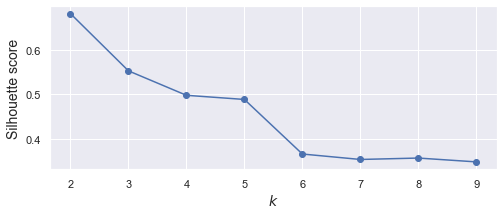Silhouette Score

### 4.1.3 Quantity of Interest: Cluster Centers

Clustering algorithms also give us important information about the location of cluster centers. To generate our cluster center information, we do the following:

# plot K-means centroids
km = KMeans(n_clusters = 3, n_init = 10)  # establish the model

# fit the data
km.fit(X);

# km centers
## KMeans(algorithm='auto', copy_x=True, init='k-means++', max_iter=300,
##        n_clusters=3, n_init=10, n_jobs=None, precompute_distances='auto',
##        random_state=None, tol=0.0001, verbose=0)
print(km.cluster_centers_)  # cluster locations
## [[ 1.2347045  -0.36280134  0.47067997 -1.02876924]
##  [ 0.78096381 -0.01849185 -1.01451835 -1.63057543]
##  [ 1.71518196 -0.1979974   1.1538938  -0.70595652]]

The array above yields three rows and four columns which tells us the coordinate location for our centroids in 4-dimensional space (remember, we have 4 features). So the first column is our X-coordinate, the second our Y-coordinate, and so on. Each row is one cluster, $$k$$, that we specified when creating our model. Since it is easier to visualize data in lower rather than higher dimensions, we plot the centroid locations below.

# predicted labels
predicted_y = km.predict(X)

# show as a square scatterplot
fig, ax = plt.subplots(figsize = (8, 8)) # set size
# plot old faithful data
plt.scatter(X[:, 0], X[:, 1], alpha = 0.8, c = predicted_y, cmap='viridis')
# plot k-means cluster centers
plt.plot(km.cluster_centers_[:, 0], km.cluster_centers_[:, 1], color = 'red', marker= 'X', markersize = 18, linewidth = 0);
# title
plt.title('Iris: K-Means Cluster Centers');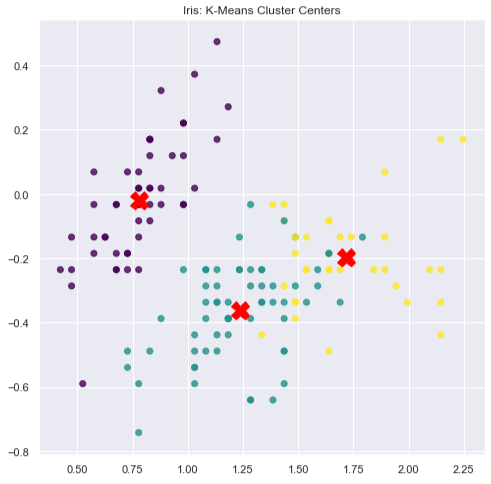K-Means Cluster Locations

## 4.2 Clustering in Practice: K-Means Drawbacks

In general, k-means is much more sensitive to outliers than other methods. Additionally, for non-spherical or oddly shaped data, Euclidean spheres of density might not be the best way to think about how your data is clustered. Lastly, the most harped-upon disadvantage of k-means clustering is that if you are exploring your data and you want to let the data tell you what the inherent structure is, you must specify your choice of $$k$$ up front. Often, there is no guidance on what $$k$$ should be and so that leads people to look for other methods for cluster analysis.

# 5 Gaussian Mixture Models (GMM)

One natural extensions of k-means clustering is Gaussian Mixture Models. GMMs, are effectively a softer fuzzy version of k-means clustering. Instead of forcing the clusters to be hard and spherical, they can be soft and have different shapes. In practice this means that each point can be assigned to a cluster with a probability between 0 and 1. The idea of a GMM is that you can have as many Gaussian models as you want, and each one has a probability in front of it that reflects how important it is to the overall model. In part, this allows GMMs to approximate any distribution, given that we use enough Gaussian components. GMMs are used for all kinds of unsupervised analysis and can particularly excel at anomaly detection.

There are four covariance_types that we must choose when modeling a GMM:

1. spherical - All clusters are spherical in shape.

2. diag - All clusters are ellipsoids but differ in shape, size, and orientation.

3. tied - All clusters have the same ellipsoid shape, size, and orientation.

4. full - The default which allows for clusters to take on any shape, size, or orientation. This choice can come at extensive computation costs.

## 5.1 GMM: Old Faithful

As we know, it turns out that not all data can be described by a single normal distribution. Instead, we can use a mixture of Gaussians to model more complex data. The graph below, depicting the famous Old Faithful data set, shows us that the data contains two distinct clusters. This means that in turn, we can model it with a two component GMM.

# load old faithful

# prepare X
X = df.values

# scale X
X = (X - X.mean()) / X.std()
# plot data points
plt.figure(figsize=(8,8))
plt.scatter(X[:, 0], X[:, 1])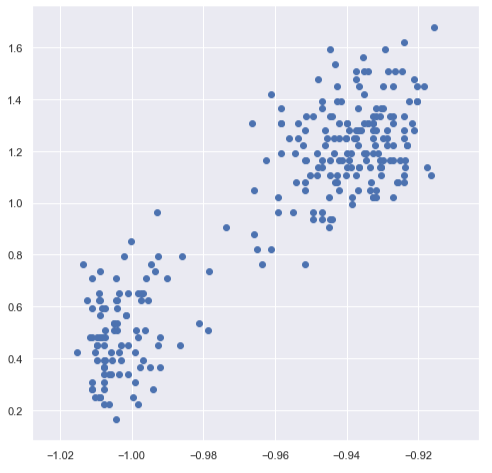Old Faithful Data Set

### 5.1.1 Quantity of Interest: AIC and BIC

Similar to the discussion above, GMMs have AIC and BIC methods built in which help us estimate the right number of clusters. As a reminder, we choose the value that is furthest left on the number line as a measure of goodness of fit.

# fit 2 component GMM
faith_gmm = GaussianMixture(n_components=2,
covariance_type='spherical',
n_init=10,
random_state=1).fit(X)

print(faith_gmm.bic(X))
## -411.5704429355076
print(faith_gmm.aic(X))
## -436.81105739957957

### 5.1.2 Instantiating the GMM

A GMM is built painstaking easy thanks to sklearn.

# fit 2 component GMM
faith_gmm = GaussianMixture(n_components=2,
covariance_type='full',
n_init=10,
random_state=1).fit(X)
# predict labels
predicted_y = faith_gmm.predict(X)

In a similar fashion to K-means, we can also plot our GMM’s centroids. Unlike the iris data set, our old faithful data is only a two dimensional data set, and so our centroid locations are easy to interpret and plot.

# gmm centroids
print(faith_gmm.means_)  # x, y
## [[-1.00292814  0.49320748]
##  [-0.93864744  1.22038157]]
# plot
plt.figure(figsize=(8,8)) # set size
# plot old faithful data
plt.scatter(X[:, 0], X[:, 1], alpha = 0.8, c=predicted_y, cmap='viridis')
# plot gmm centroid
plt.plot(faith_gmm.means_[:, 0], faith_gmm.means_[:, 1], color = 'red', marker= 'X', markersize = 18, linewidth = 0);
# title
plt.title('Old Faithful: GMM Cluster Centers');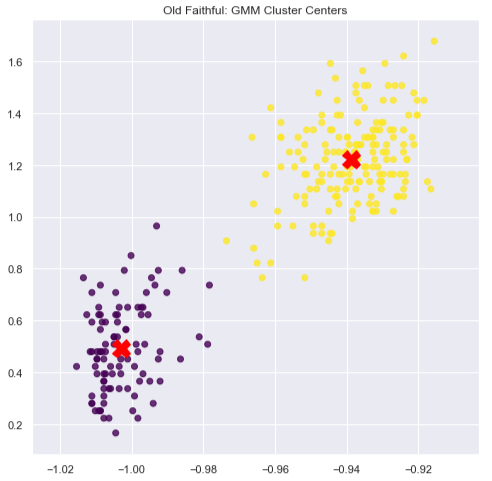Old Faithful: GMM Cluster Locations

## 5.2 GMM: Anomaly Detection

GMMs excel at detecting anomalies because of the way it draws density contours around its cluster mean. This is in part done through score_samples() which yields the natural log of the probability density function for each observation. As the score increases, the PDF’s density increases as well. We can use these quantities of interest to identify anomalies given our specified threshold.

In the code below, we identify two observations, one outlier and one inlier and show the natural log of the probability density function. Notice that observations located further and further away from the cluster have an increasingly negative value.

# outlier
outlier = X
outlier_log_pdf = faith_gmm.score_samples(outlier.reshape(1, -1))
print(outlier_log_pdf)
## [-1.57586764]
# inlier
inlier = X
inlier_log_pdf = faith_gmm.score_samples(inlier.reshape(1, -1))
print(inlier_log_pdf)
## [3.60998235]

Next, we specify our outlier threshold of 4% (or any that we like). density_threshold returns the value of the natural log of the probability density function that we will use to identify anomalous observations.

# anomaly
densities = faith_gmm.score_samples(X)
density_threshold = np.percentile(densities, 4)  # 4% threshold for outliers
print(density_threshold)
## 0.5657045790858946
anomalies = X[densities < density_threshold]  # filter anomalous data

Lastly, we can plot our anomalous data and observe that the observations that it selects are indeed far from our cluster centers.

# instantiate plot
plt.figure(figsize=(8, 8))
# plot gmm centroid
plt.plot(faith_gmm.means_[:, 0], faith_gmm.means_[:, 1], color = 'red', marker= 'X', markersize = 18, linewidth = 0);
# plot data
plt.scatter(X[:, 0], X[:, 1], alpha = 0.8, c = predicted_y, cmap='viridis')
# plot anomalies
plt.scatter(anomalies[:, 0], anomalies[:, 1], color='r', marker=',', s = 12)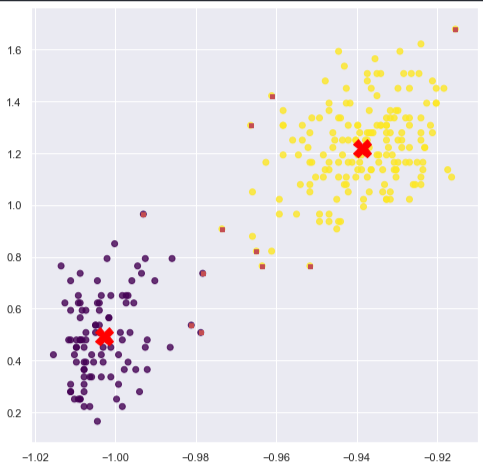Old Faithful: Anomalous Observations at the 4% Level

# 6 Sources

1. Géron, Aurélien. Hands-On Machine Learning with Scikit-Learn, Keras, and TensorFlow: Concepts, Tools, and Techniques to Build Intelligent Systems. O’Reilly Media, 2019. https://github.com/ageron/handson-ml2/blob/master/09_unsupervised_learning.ipynb